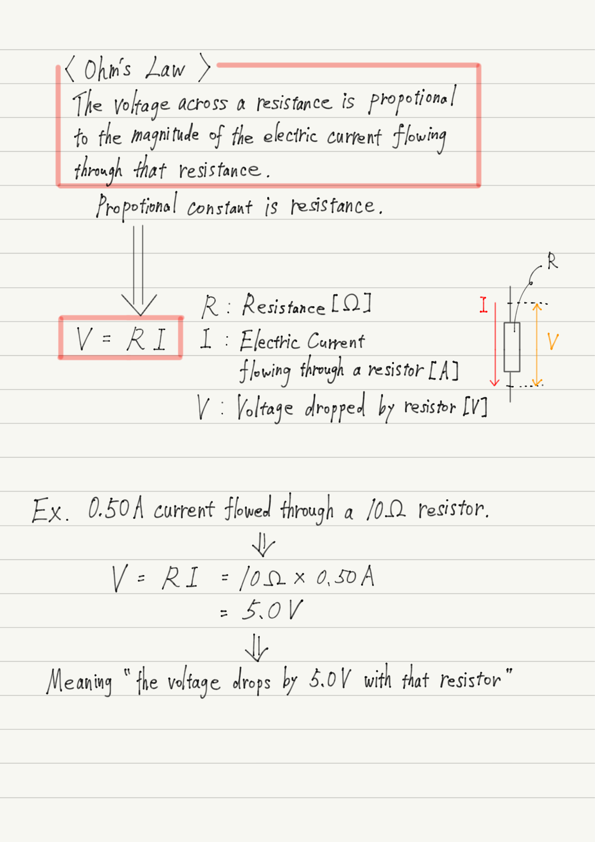# Ohm’s Law

Thank you for your patience. Now it is time to explain the contents of Ohm’s law.
Ohm’s law is a law that describes the relationship between electric current, voltage, and resistance.

## Meaning of Ohm’s Law

Ohm’s law is expressed by the equation “V=RI “.
What does this equation mean?
Look closely at this equation. Does it resemble anything?

Yes, It has the same form as the proportionality equation “y=ax ” we learned in math!

The meaning of y=ax is x and y are proportional and their proportionality constant is a .

Applying this to Ohm’s law V=RI as it is, we get I(Electric Current) and V(Voltage) are proportional and their proportionality constant is R(Resistance)”.

Ohm’s law should be understood properly in this way, rather than by memorizing the equation.

## What do we assign to each symbol?

I and V appear in the Ohm’s law equation, but in textbooks they are simply written as “Electric Current” and “Voltage”. This is an oversimplification.
Those who view V simply as a voltage tend to assign the battery voltage to V.
However, that is a mistake.

Now let me tell you the exact wording. First, R. This is the value of the resistance.

Next is I, which is “the magnitude of the electric current flowing through that resistance”.

Finally, V. This is “how much the voltage drops with that resistor”.
Note that battery voltage has nothing to do with Ohm’s law!

The function of a resistor in a circuit was to reduce the voltage. If you have forgotten, please refer to the previous article.

https://www.yukimura-physics.com/entry/elemag-f06

Ohm’s law shows the relationship between the “falling voltage” and the “the magnitude of the flowing electric current”.

## Summary of this lecture## Next Time

In this lecture, we have summarized the contents and formulas of Ohm’s law, but what is important is its usage.
In the next lecture, I will explain how Ohm’s law can be used in actual circuit problems.

https://www.yukimura-physics.com/entry/elemag-f08

error: Content is protected !!